Velocity-Time(s-t)Graph

# Our Objective

To plot the velocity–time (v – t) graph for an object moving with uniform accelerations from a given set of v – t data and to determine the acceleration of the moving object and the distance moved by the object.

# The Theory

An object is said to be in motion if it changes its position with time, with respect to its surroundings. The length of actual path travelled by the object in motion in a given time is known as distance travelled by the object.

Different objects may take different amounts of time to cover a given distance.  We can determine how fast or how slow an object is moving by calculating the speed of the object. Speed of an object is the distance travelled by the object in unit time.As the SI unit for distance is meters and time is in seconds, the SI unit of speed is metre per second. This is represented by the symbol ms-1 or m/s. The other units of speed include centimetre per second (cm s-1) and kilometre per hour (km h-1).

The speed of an object moving in a definite direction is known as its velocity. The velocity of an object can be uniform or variable. It can be altered by changing the object’s speed, direction of motion or both.

## Uniform motion

When an object travels equal distances in equal intervals of time, it is said to be in uniform motion. During uniform motion of an object along a straight line, the velocity remains constant with time.

## Non- uniform Motion

Motions where objects cover unequal distances in equal intervals of time are referred as non-uniform motion. For non-uniform motion, the velocity of the object varies with time. It has different values at different instants and at different points of the path. The change in velocity of an object per unit time is known as acceleration.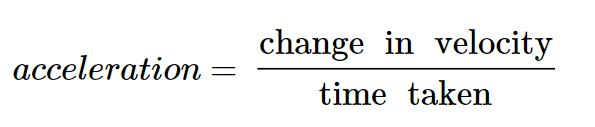If an object moving with an initial velocity u (at time 0) attains the final velocity v (in time t), then the acceleration a is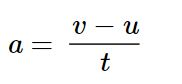If the acceleration is in the same direction as the velocity, it is considered positive; otherwise, it is considered negative. The SI unit of acceleration is m s-2.

## Velocity - time graph

A velocity-time graph is the graphical representation of change in velocity of an object with time. Conventionally, the x-axis represents time, and the y-axis represents velocity of the object.

## Slope of the v-t graph

The slope of a line is a measure of its steepness. The slope is defined as the ratio of the vertical change (change in y-axis) between two points, to the horizontal change (change in x-axis) between the same two points. If we consider two points, (x1, y1) and (x2, y2) on the given line, then the slope,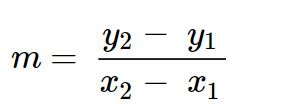For the velocity-time graph the slope of the graph is the ratio of change in velocity of the object to the corresponding time interval. This gives as the acceleration of the object. Assuming two points (t1, v1) and (t2, v2), the slope or acceleration of object,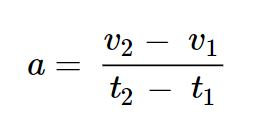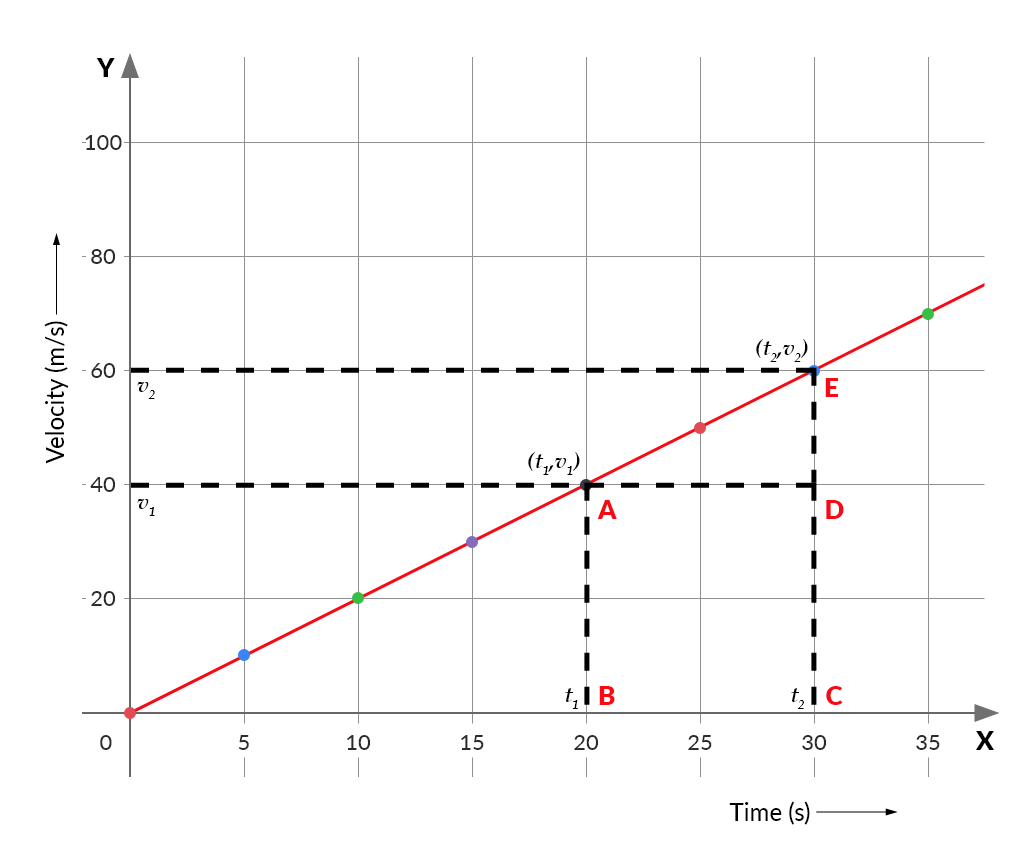If the acceleration of the object remains same at all instants of time then the object is said to be in uniformly accelerated motion. Thus the velocity-time graph of a uniformly accelerated object will be a straight line. The slope of v-t graph can give a comparison of the acceleration of different moving objects.

## Area under the v-t graph

We know that distance travelled by an object is given by the product of velocity and time. Hence, for a given time interval, the area under the velocity-time graph gives the distance moved by the object in that time interval. The distance s travelled by the car in a time interval t2 – t1 will be given by the area ABCDE under the velocity-time graph. That is,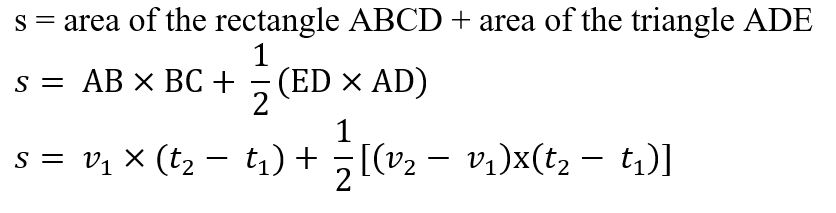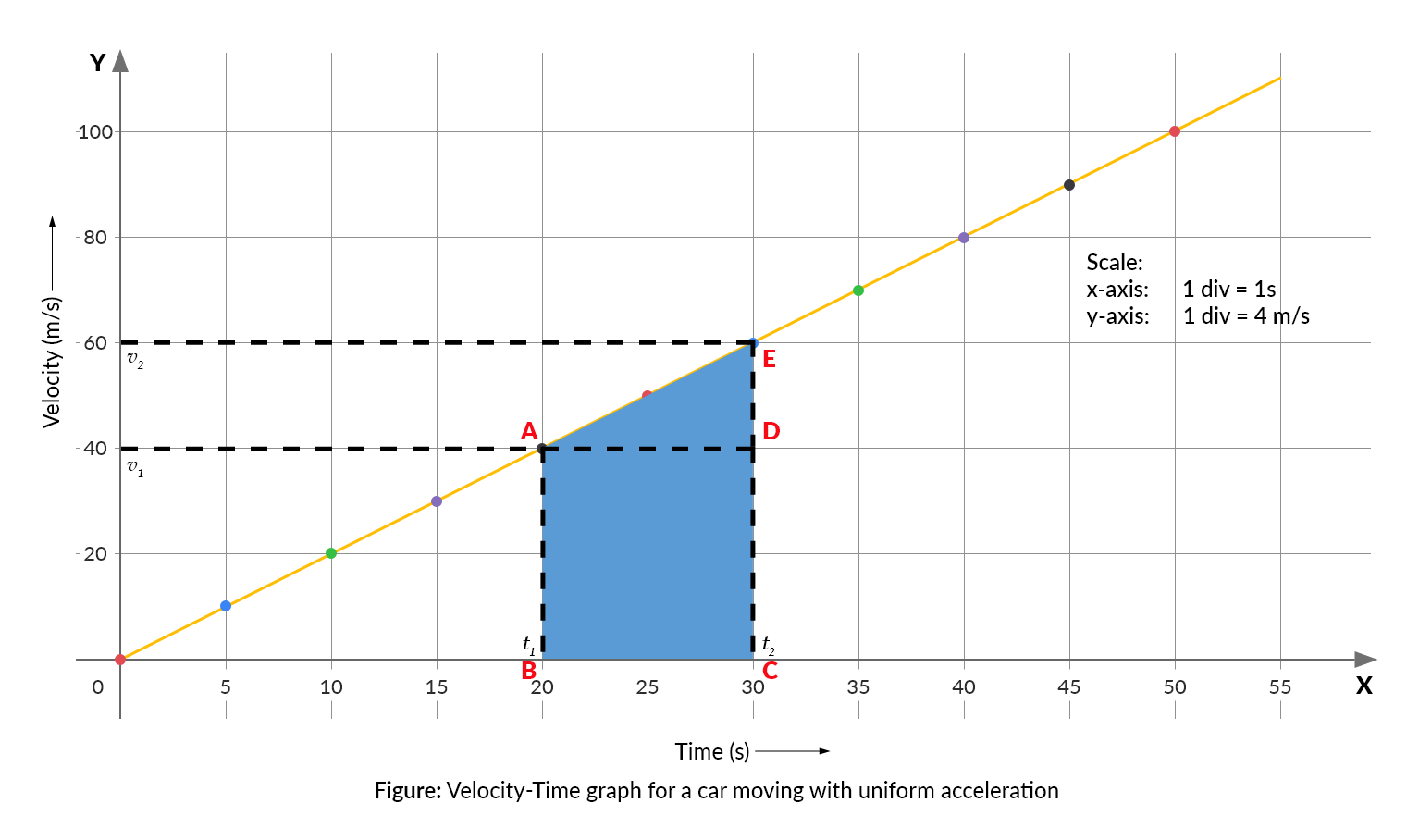## Some examples of Velocity - Time graph

• An object in uniform motion

Speed of such an object is constant. Hence, the velocity- time graph for an object in uniform motion is a straight line parallel to the time axis. As the change in velocity of the object for any time interval is zero, the slope of the graph is zero.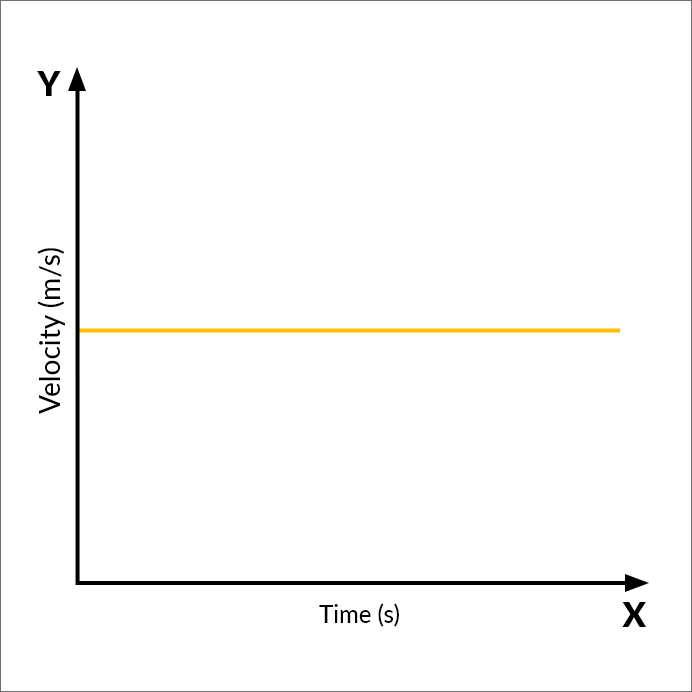• An object in uniform acceleration

For a uniformly accelerated object, the velocity of the object increases with time. Since the acceleration of the object remains same at all instants of time, the slope of the v-t graph will also be constant. The velocity-time graph of a uniformly accelerated object will therefore be a straight line inclined to time axis. The nature of the graph shows that the velocity changes by equal amounts in equal time intervals. As a result, the velocity of the object is directly proportional to the time.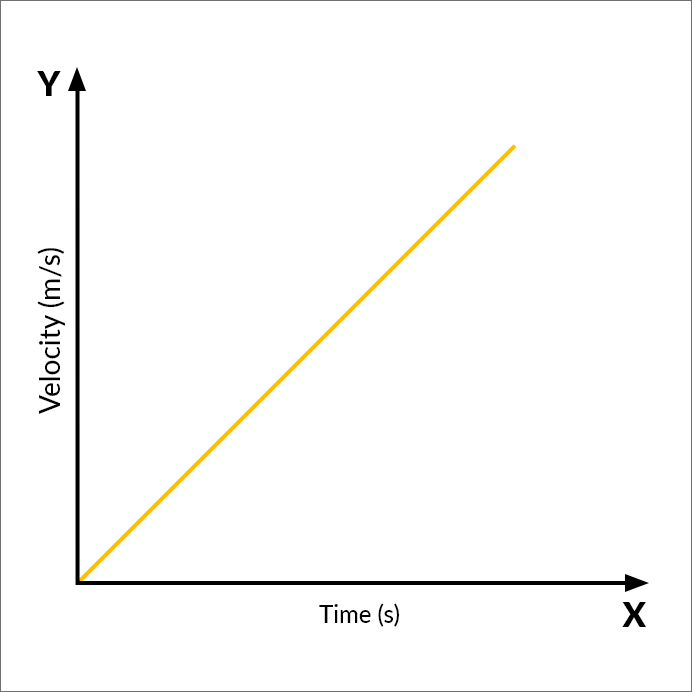• An object in non-uniform acceleration

Velocity-time graph for such a motion is represented in the figure below. In this case, the acceleration of an object changes with time. The slope of the v-t graph for object in non-uniform acceleration is different at different points under consideration. If the acceleration of the object increases, the slope of the graph increases. In the graph given, the acceleration of the object between the points B and C is greater than the acceleration of object between A and B. The rate of increase in velocity is more between B and C which is indicated by the steeper slope. On the other hand, between the points C and D, the velocity of the object decreases with time. The acceleration of object between C and D is negative.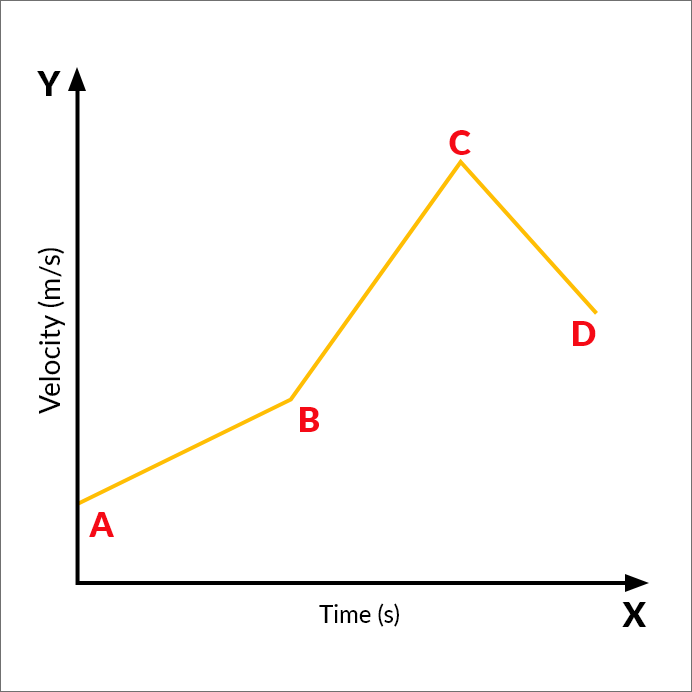## APPLICATIONS

• The slope of a v – t graph is a measure of acceleration of the moving object. Given different v – t graphs for different moving objects, we can make a comparison of the acceleration of the objects by determining their slopes. Larger the slope of the graph higher is the acceleration of the moving object.
• The area under v-t graph gives the distance travelled. Hence, an object with a larger area under the v-t graph will have travelled a greater distance.
• The v – t graph plotted here shows the motion of the object for a given range of time interval. Using this graph, one can even determine the velocity (or acceleration) at a time beyond the range of time intervals given in the data. This can be done by extending the line graph to estimate the unknown values, a method called as extrapolation of the graph. For this, the graph should be extrapolated.

# Learning Outcomes

• Students learn to plot velocity – time graph of an object from the given data.
• Students develop knowledge of how physical quantities like velocity and time are represented graphically.
• Students learn to find the slope of the graph.
• Students comprehend the relationship between an object's acceleration, velocity, and time. They learn to employ this concept in finding acceleration of the object from the velocity – time graph.
• Students discover the relationship between the area under the velocity-time graph and the distance travelled by an object.
• Students learn to distinguish the velocity – time graph of uniform motion, uniform acceleration and non-uniform acceleration.
• Students gain the ability to infer information from a graph.